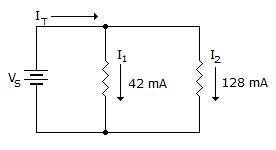# Electronics - Parallel Circuits - Discussion

### Discussion :: Parallel Circuits - Filling the Blanks (Q.No.11)

11.If R1 opens in the given circuit, the total current equals ___.

 [A]. 86 mA [B]. 170 mA [C]. 42 mA [D]. 128 mA

Explanation:

No answer description available for this question.

 Govind said: (Sep 22, 2011) According to kcl law I=I1+I2;. Whn open i1=0; hence total current is 128mA.

 Smiley said: (Aug 17, 2013) But when i1 is open then total current will flow through R2 so it should be 170 isn't it ?

 Vignesh said: (Jul 30, 2014) Yes I have same doubt, But when i1 is open then total current will flow through R2 so it should be 170 isn't it ?

 Naisy said: (Sep 16, 2015) I don't understand the reason the correct answer is 128 mA, since I1 is closed, meaning the total current will flow to I2 which makes it 170 mA. IT = I1+I2 and if I1 is closed IT = I2.

 Siddharth said: (Jan 10, 2016) The current flow across the resister depends on the value of the individual resister when they connected in parallel assume r1 as 1 ohm. And r2 as 1 ohm and and we as 1V than Rt will be 0.5 ohm. It will be 2A, so if r2 removed then It = 1V \r1 = 1v/1ohm = 1A. So according to ohms law the current flow across r1 is 1A only even though total circuit current is 2A. So when n resisters connected in parallel current flow depends on the value of resister only.

 Dra said: (Apr 1, 2016) Need resistance for current to flow, if it is open the current cannot flow through the open resistor.

 Waheed Ktk said: (Nov 8, 2016) The Correct option is 170 A because the whole current will pass through R2.

 Abhas said: (Aug 10, 2017) Current always flow through minimum resistance path 170mA.